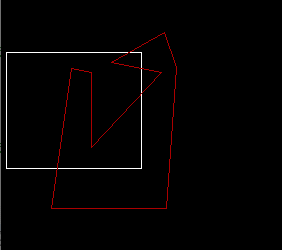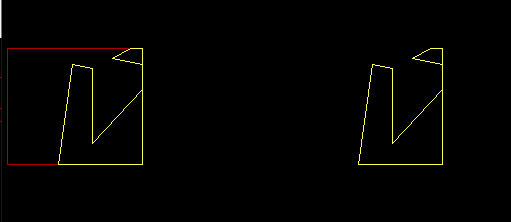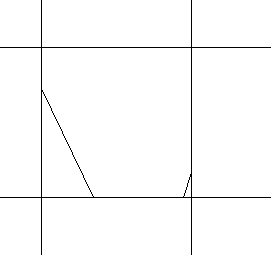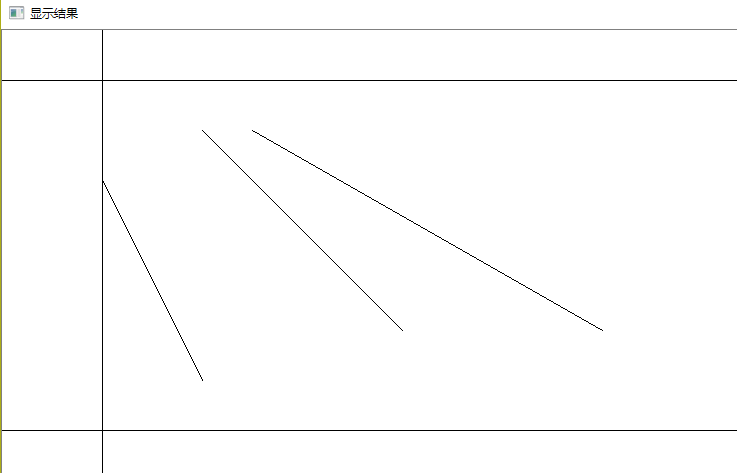• 计算机图形学(简单多边形裁剪算法).doc
2021-07-29 01:11:41

计算机图形学(简单多边形裁剪算法)

简单多边形裁剪算法

摘要：多边形裁剪算法与线性裁剪算法具有更广泛的实用意义，因此它是目前裁剪研究的主要课题。本文主要介绍了一种基于多边形顶点遍历的简单多边形裁剪算法，它有效降低了任意多边形裁剪复杂度。通过记录交点及其前驱、后继信息，生成结果多边形，该算法简化了交点的数据结构，节省了存储空间，降低了算法的时间复杂度，具有简单、易于编程实现、运行效率高的特点。

关键词：多边形裁剪；交点；前驱；后继；矢量数组

一、技术主题的基本原理

简单多边形裁剪算法综合考虑现有多边形裁剪算法的优缺点，它是一种基于多边形顶点遍历来实现简单多边形裁剪工作的。其主要的原理是遍历多边形并把多边形分解为边界的线段逐段进行裁剪，输出结果多边形。

二、发展研究现状

int nPredecessorlndex=0／／前驱序号

int nSuccessorIndex=0／／后继序号

}

说明：CrossPointIndex用于记录交点在多边形中的前驱与后继的序号信息，以及记录同一交点在两个多边形中顶点序号。即若P为多边形S与多边形C的交点，为了表示P在S和C中为同一点，则可用CrossPointIndex记录用nPredecessorIndex记录P在S中的序号、用nSuccessorIndex记录P在C中序号。

3)线段的数据结构如下：

Segment{

int nStartIndex=0

int nEndIndex=0

int* pIndexes;

int nIndexCount;

}

说明：Segment表示多边形在另一个多边形内(外)的线段，nStartaIndex为Segment起始顶点的序号，nEndIndex为Segment终止顶点的序号，pIndexes为起始顶点与终止顶点之间的顶点序号集合，nIndexCount为pIndexes中元素个数。

3、算法设计

1)第一阶段：

采用射线法计算并判断S(或C)在C(或S)内，并修改S(或C)顶点Vertex的IsInPolygon的值。

由于射线可以任意选取，为了方便可以将射线定为从被测点开始射向X轴坐标正方向的水平射线。由于多边形的一条边与射线的交点最为1个，因此可以循环遍历每条边并判断边与射线有无交点，若有交点，计数器加1,。最后得到的计数器的值即多边形与射线的交点个数。若交点个数为奇数，则被测点在多边形内部，若交点个数为偶数，则被测点在多边形外部。对于多边形的任意一条边，为了尽量避免求交点时用到乘除法，将判断该边与射线有无交点的算法可以包含3步：

判断边的两个顶点是否均在被测点左方或者下方或者上方，若是则无交点，返回假并退出；

若不满足第一条原则，且满足两个顶点均在被测点右方，则一定有顶点，返回真并退出；

若以上两条都不满足，求出边与射线的交点，比较交点的X坐标与被测点的X坐标，若前者大于后者则返回真并退出，否则返回假并退出。

设边的两个顶点坐标分别为(x1,y1)和(x2,y2),则边的直线方程可写为：

X=m(y-y1)+x1

其中，m=(x2-x1)/(y2-y1)为直线斜率的倒数。使用该方程时需要两次乘除法，效率较低。为了尽量避免求交点，第三部可以采用二分法将边分成两段，对其中两个端点分别跨过射线两侧的边段重新进行以上第一步和第二步的判断，若以上两步中还没有推出，再继续二分，直到能够被第一步和第二步判断并退出。采用二分法则避免了乘除法，算法中只有除2运算和一些判断，适合于硬件处理，二分法的循环次数一般较少，当被测点位于边上时，循环次数组最多。

其具体的算法如下：(Point为被测点变量，point1、point2为一条边的两个端点变量)

If(piont2.y

{

P1=point2;

p2=point1

}

Else

{

p1=point1;

p2=point2;

}

if(p1.y>point.y||p2.y

Return false;//无交点

Else If(p1.x

Return false ；无交点

Else if (p1.x>point.x&&p2.x>point.x)Return

更多相关内容
• 多边形裁剪算法，还不错，学到挺多的，共享给大家看看
• 已经处理退化的多边形裁剪算法 //编译环境：Visual C++ 6.0，EasyX_20190219(beta) #include<graphics.h> #include<conio.h> #include<iostream> #define max 30 using namespace std; //设置...

已经处理退化边的多边形裁剪算法

//编译环境：Visual C++ 6.0，EasyX_20190219(beta)
#include<graphics.h>
#include<conio.h>
#include<iostream>
#define max 30
using namespace std;

//设置裁剪框的大小和位置,裁剪多边形顶点和顶点数，以全局变量给出
double xl=5,xr=140,yt=190,yb=74;
int inlength=9;
POINT Vertex[]={ {110,84},{160,94},{90,169},{90,94},{70,90},{50,230}, {165,230},{175,89},{163,54} };
//求多边形的一条边sp和裁剪边point0 point1的交点
void Intersect(POINT S,POINT P,POINT point0,POINT point1,POINT &I)
{
if(point0.y==point1.y)//水平裁剪边
{
I.y=point0.y;
I.x=S.x+(point0.y-S.y)*(P.x-S.x)/(P.y-S.y);
}
else//竖直裁剪边
{
I.x=point0.x;
I.y=S.y+(point0.x-S.x)*(P.y-S.y)/(P.x-S.x);
}
}

//测试顶点与裁剪边的内外关系
bool Inside(POINT text,POINT point0,POINT point1)
{
if(point1.x>point0.x){//裁剪边为窗口的下边
if(text.y>=point0.y)
return true;}

else if(point1.x<point0.x){//裁剪边为窗口的上边
if(text.y<=point0.y)
return true;}

else if(point1.y>point0.y){//裁剪边为窗口的右边
if(text.x<=point0.x)
return true;}

else if(point1.y<point0.y){//裁剪边为窗口的左边
if(text.x>=point0.x)
return true;}

return false;
}

//将新的定点加入结果多边形顶点表
void Output(POINT newpoint,int &length,POINT outps[])
{
outps[length].x=newpoint.x;
outps[length].y=newpoint.y;
length++;
}

//裁剪算法
void SutherlandHodgmanPolygonClip(int inlength,POINT inpoints[],int &outlength,POINT outpoints[],POINT point0,POINT point1)
{
POINT S,P,I;
int j;
outlength=0;
S=inpoints[inlength-1];
for(j=0;j<inlength;j++)
{
P=inpoints[j];
if(Inside(P,point0,point1))
{
if(Inside(S,point0,point1))
{Output(P,outlength,outpoints);}
else
{
Intersect(S,P,point0,point1,I);
Output(I,outlength,outpoints);
Output(P,outlength,outpoints);}}
else if(Inside(S,point0,point1))
{
Intersect(S,P,point0,point1,I);
Output(I,outlength,outpoints);}
S=P;}
}

int main()
{
POINT Edge[]={ {xr,yb},{xr,yt},{xl,yt},{xl,yb} };
initgraph(1000,900);
polygon(Edge,4);
setcolor(RED);
polygon(Vertex,inlength);
int i;
POINT OutPts1[max],OutPts2[max],OutPts3[max],OutPts4[max];
int length1,length2,length3,length4;
SutherlandHodgmanPolygonClip(inlength,Vertex,length1,OutPts1,Edge,Edge);//右边窗口裁剪边
SutherlandHodgmanPolygonClip(length1,OutPts1,length2,OutPts2,Edge,Edge);//下边窗口裁剪边
SutherlandHodgmanPolygonClip(length2,OutPts2,length3,OutPts3,Edge,Edge);//左边窗口裁剪边
SutherlandHodgmanPolygonClip(length3,OutPts3,length4,OutPts4,Edge,Edge);//上边窗口裁剪边
MOUSEMSG m;
while(true)
{
m=GetMouseMsg();
switch(m.uMsg)
{
case WM_LBUTTONDOWN:
cleardevice();
setcolor(RED);
polygon(Edge,4);
setcolor(YELLOW);
polygon(OutPts4,length4);
for(i=0;i<length4;i++)
{OutPts4[i].x+=300;}
polygon(OutPts4,length4);
break;
case WM_RBUTTONDOWN:
return 0;
}
}
_getch();
closegraph();
return 0;
}以上是按照右下左上的顺序进行裁剪的，当然读者可以按照自己的习惯进行调整。

展开全文• 算法的基本思想是每次用窗口的一条边界及其延长线来裁剪多边形的各。多边形通常由它的顶点序列来表示，经过裁剪规则针对某条边界裁剪后，结果形成新的顶点序列，又留待下条边界进行裁剪，…，直到窗口的所有边界...计算机图形学
• Sutherland-Hodgman算法也叫逐边裁剪法，该算法是萨瑟兰德(I．E．Sutherland)和霍德曼(Hodgman)在1974年提出的。这种算法采用了分割处理、逐边裁剪的方法。一，基本思想: 一次用窗口的一条边裁剪多边形。 考虑窗口的...

Sutherland-Hodgman算法也叫逐边裁剪法，该算法是萨瑟兰德(I．E．Sutherland)和霍德曼(Hodgman)在1974年提出的。这种算法采用了分割处理、逐边裁剪的方法。一，基本思想: 一次用窗口的一条边裁剪多边形。 考虑窗口的一条边以及延长线构成的裁剪线该线把平面分成两个部分:可见一侧；不可见一侧。多边形的各条边的两端点S、P。它们与裁剪线的位置关系只有四种情况（1）仅输出1个顶点P；情况（2）输出0个顶点；情况（3）输出线段SP与裁剪线的1个交点I；情况（4）输出线段SP与裁剪线的1个交点I和1个终点P**

顺时针访问多边形各条边，可以以上面的基本思想来对上图多边形进行裁剪，可以得到正确的结果二、算法实现：　　1、已知：多边形顶点数组src，顶点个数n，
定义新多边形顶点数组dest。　　2、赋初值：用变量flag来标识：
0表示在内侧，1表示在外侧。 3、对多边形的n条边进行处理，对当前点号的考虑为：0～n-1。
for(i=0；i<n；i++)
{
if(当前第i个顶点是否在边界内侧？) {
if(flag!=0) /前一个点在外侧吗？/
{
flag=0；/从外到内的情况，将标志置0,作为下一次循环的前一点标志/
(dest + j) =求出交点；　/将交点dest放入新多边形/ j++；
}

(dest + j)= (src + i)； /将当前点srci放入新多边形/ j++；
}
else
{
if(flag==0) /前一个点在内侧吗？/
{
flag=1；/从内到外的情况，将标志置1,作为下一次循环的前一点标志/
(dest + j) =求出交点；　/将交点dest放入新多边形/ j++；
}
}
s= (src + i)； /将当前点作为下次循环的前一点/
}三，算法特点：　　Sutherland－Hodgeman多边形裁剪算法具有一般性，被裁剪多边形可以是任意凸多边形或凹多边形，裁剪窗口不局限于矩形，可以是任意凸多边形。
上面的算法是多边形相对窗口的一条边界进行裁剪的实现，对于窗口的每一条边界依次调用该算法程序，并将前一次裁剪的结果多边形作为下一次裁剪时的被裁剪多边形，即可得到完整的多边形裁剪程序。//点在有向线段那侧/向量叉积法 　　为简单计，测试点表示为P点。假设窗口边界方向为顺时针，如图中所示，对于其中任一边界向量，从向量起点A向终点B看过去： 　　如果被测试点P在该边界线右边(即内侧)，AB×AP的方向与X-Y平面垂直并指向屏幕里面，即右手坐标系中Z轴的负方向。 　　反过来，如果P在该边界线的左边(即外侧)，这时AB×AP的方向与X-Y平面垂直并指向屏幕外面，即右手坐标系中Z轴的正方向。 　　设：点P(x，y)、点A(xA，yA)、点B(xB，yB)， 　　　　向量AB={(xB-xA)，(yB-yA)}， 　　　　向量AP={(x-xA)，(y-yA)}， 　　那么AB×AP的方向可由下式的符号来确定： V=(xB-xA)·(y-yA)-(x-xA)·(yB-yA) // V为AB叉乘AP的结果向量中的z分量 　　因此，当V≤0时，P在边界线内侧； 　　　　而V>0时，P在边界线外侧。/typedef point{ int x; int y;}RtPoint; typedef struct{ RtPoint sp; //裁剪窗口边界向量起点 RtPoint ep; //裁剪窗口边界向量终点}_RtInSide; static int _RtInSide(RtVector vector, RtPoint point) // 计算上面的V，vector为裁剪窗口边界向量，point为上面的p点{ return (vector.ep.x - vector.sp.x) * (point.y - vector.sp.y) - (vector.ep.y - vector.sp.y) * (point.x - vector.sp.x);} //多边形点必须是顺时针方向// src保存的是裁剪窗口坐标点，应该是顺时针方向保存，num为裁剪窗口边界数量，dest保存裁剪后的多边形顶点数组，total为dest大小int rtPrunePSH(RtPoint src, int num, RtPoint** dest, int* total){ int i = 0, j = 0, k = -1, flag = 0; RtPoint start, stop;//被剪裁多边形的边向量起点和终点 RtPoint sp, ep;//剪裁窗口边界向量的起点和终点 RtPoint* temp = NULL; // temp保存针对某一裁剪窗口边界裁剪后的新坐标 temp = (RtPoint*)malloc(sizeof(RtPoint) * 3 * (*total)); if (temp == NULL) return -1; sp = *(src + num - 1); for ( i = 0; i < num; i++)//剪裁窗口 { ep = *(src + i); start = *((*dest) + *total - 1); flag = _RtInSide(rtVector(sp, ep), start) > 0 ? 0 : 1; //在外侧，flag为0，在内侧时flag为1 for ( j = 0; j < *total; j++)//被剪裁的多边形 { stop = *((*dest) + j); if (_RtInSide(rtVector(sp, ep), stop) <= 0)//当前第i个顶点是否在边界内侧 { if (flag == 0)/前一个点在外侧吗？/ { flag = 1;/从外到内的情况，将标志置1,作为下一次循环的前一点标志/ k++; CRtPoint point; CRtPoint st(sp.x, sp.y), et(ep.x, ep.y); CRtLine v1(st, et);//v1为由窗口边界向量起点sp和终点ep组成的直线 st.SetData(start.x, start.y); et.SetData(stop.x, stop.y); //v2为由多边形当前边起点start和终点stop组成的直线 CRtLine v2(st, et); v2.Intersect(v1,point); //两直线求交运算，交点保存到point数组里面 (temp + k)->x = point;/将交点放入新多边形/ (temp + k)->y = point; } k++; *(temp + k) = stop;/将当前点pi放入新多边形/ } else { if (0 != flag)/前一个点在内侧吗？/ { flag = 0;/从内到外的情况，将标志置1,作为下一次循环的前一点标志/ k++; CRtPoint point; // 同上面一样 CRtPoint st(sp.x, sp.y), et(ep.x, ep.y); CRtLine v1(st, et); st.SetData(start.x, start.y); et.SetData(stop.x, stop.y); CRtLine v2(st, et); v2.Intersect(v1,point); (temp + k)->x = point;/将交点放入新多边形/ (temp + k)->y = point; } } start = stop;/将当前点作为下次循环的前一点/ } sp = ep; *total = k + 1; // k+1为一次裁剪后生成的新坐标点的数量 memcpy(*dest, temp, sizeof(RtPoint) * (*total)); k = -1; } return 0;}
转载来源：http://blog.csdn.net/juyingmin/article/details/5611458
https://blog.csdn.net/damotiansheng/article/details/43274183

展开全文• ## 裁剪算法

千次阅读 2018-03-05 14:00:36
Cohen-SutherLand算法 算法理论 算法总体思想是，对于每条线段P1P2P1P2P_1P_2，(1)若P1P2P1P2P_1P_2全在窗口内，取之；(2)若P1P2P1P2P_1P_2明显在窗口外，弃之；(3)若无法满足上述两条件，则把线段分为两段，...

# Cohen-SutherLand算法

## 算法理论

算法总体思想是，对于每条线段 P1P2 P 1 P 2 $P_1P_2$，(1)若 P1P2 P 1 P 2 $P_1P_2$全在窗口内，取之；(2)若 P1P2 P 1 P 2 $P_1P_2$明显在窗口外，弃之；(3)若无法满足上述两条件，则把线段分为两段，窗口外的可弃之，剩下的重复处理。
为方便处理，将图形区按下表分类：

100110001010
000100000010
010101000110

于是每个点可以根据上图获得一个区域码。对于某个线段，若：
code1=code2=0，则线段在窗口内
(code1&code2)!=0，则线段在窗口的同侧，线段在窗口外
否则即为第三种情况。则将窗口外的端点与边界求交点，替换原有交点，继续计算。

中点分割算法的理论与上述算法基本类似，就是求线段端点的方法不同，使用二分法寻找边界点，而非直接计算。

## 算法实现

#include<iostream>
#include<algorithm>
#include<opencv2\core\core.hpp>
#include<opencv2\highgui\highgui.hpp>
#include"MyLine.h"

#define LEFT 1
#define RIGHT 2
#define BOTTOM 4
#define TOP 8
#define WIDTH 1000
#define HEIGHT 500

using namespace std;
using namespace cv;

int xl, xr, yb, yt;

void DDALine(Mat& m, const int x0, const int y0, const int x1, const int y1, const cv::Vec3b& v);
int Encode(int x, int y);
void Cohen_SutherLand(Mat& m, MyLine& L);

int main(){
vector<MyLine> lv;
int n,i;
int x1, y1, x2, y2;
cout << "please input the number of lines" << endl;
cin >> n;
for (i = 1; i <= n; i++){
cout << "please input the coordinates of the vertexes of the No." << i << " line" << endl;
cin >> x1 >> y1 >> x2 >> y2;
lv.push_back(MyLine(MyPoint(x1, y1), MyPoint(x2, y2)));
}
cout << "please input the border of the screen, xl, xr, yb, yt" << endl;
cin >> xl >> xr >> yb >> yt;
Mat imageROI = Mat(HEIGHT, WIDTH, CV_8UC3, Scalar(255, 255, 255));
for (i = 0; i < HEIGHT; i++){
imageROI.at<Vec3b>(i, xl) = Vec3b(0, 0, 0);
imageROI.at<Vec3b>(i, xr) = Vec3b(0, 0, 0);
}
for (i = 0; i < WIDTH; i++){
imageROI.at<Vec3b>(yb, i) = Vec3b(0, 0, 0);
imageROI.at<Vec3b>(yt, i) = Vec3b(0, 0, 0);
}//draw border
//Cohen-SutherLand
vector<MyLine>::iterator iter;
for (iter = lv.begin(); iter != lv.end(); iter++){
Cohen_SutherLand(imageROI, *iter);
}
namedWindow("显示结果");
imshow("显示结果", imageROI);
waitKey();
}

int Encode(int x, int y){
int code=0;
if (x < xl){
code = code | LEFT;
}
else if (x>xr){
code = code | RIGHT;
}
if (y < yb){
code = code | BOTTOM;
}
else if (y>yt){
code = code | TOP;
}
return code;
}

void Cohen_SutherLand(Mat& m, MyLine& L){
int code1, code2, code;
float x, y, x1, y1, x2, y2;
x1 = L.getP1().GetX();
y1 = L.getP1().GetY();
x2 = L.getP2().GetX();
y2 = L.getP2().GetY();
code1 = Encode(x1, y1);
code2 = Encode(x2, y2);
while (code1 != 0 || code2 != 0){
if ((code1&code2) != 0){
return;
}
code = code1;
if (code == 0){
code = code2;
}
if ((LEFT&code) != 0){
x = xl;
y = y1 + (y2 - y1)*(xl - x1) / (x2 - x1);
}
else if ((RIGHT&code) != 0){
x = xr;
y = y1 + (y2 - y1)*(xr - x1) / (x2 - x1);
}
if ((BOTTOM&code) != 0){
y = yb;
x = x1 + (x2 - x1)*(yb - y1) / (y2 - y1);
}
else if ((TOP&code) != 0){
y = yt;
x = x1 + (x2 - x1)*(yt - y1) / (y2 - y1);
}
if (code == code1){
x1 = x;
y1 = y;
code1 = Encode(x, y);
}
else{
x2 = x;
y2 = y;
code2 = Encode(x, y);
}
}
DDALine(m, x1, y1, x2, y2, Vec3b(0, 0, 0));
return;
}

结果：算法总体比较简单，就是!=与&的优先级需要注意一下。

# Liang-Barskey算法

## 算法理论

首先需要介绍Cyrus-Beck算法，可以对多边形区域进行裁剪。对于某线段，用参数方程表示为:

P(t)=(P2P1)t+P1 P ( t ) = ( P 2 − P 1 ) t + P 1
对于凸多边形上的任意一点有：
N(P(t)A)>0 N ∙ ( P ( t ) − A ) > 0
则线段必定通过多边形的内侧。其中N是该点法向量。
故可见线段的参数区间为：
{N(P(t)A)>00t1 { N ∙ ( P ( t ) − A ) > 0 0 ⩽ t ⩽ 1
实际计算时，只需要求出 tmin t m i n $t_{min}$ tmax t m a x $t_{max}$，即可求出线段的两端点。上式可变为：
{N((P2P1)t+P1A)>00t1 { N ∙ ( ( P 2 − P 1 ) t + P 1 − A ) > 0 0 ⩽ t ⩽ 1
{N(P1A)+N(P2P1)>00t1 { N ∙ ( P 1 − A ) + N ∙ ( P 2 − P 1 ) > 0 0 ⩽ t ⩽ 1
N(P2P1)=0 N ∙ ( P 2 − P 1 ) = 0 $N\bullet (P_2-P_1)=0$时，线段与对应边平行，当 N(P1A)>0 N ∙ ( P 1 − A ) > 0 $N\bullet (P_1-A)>0$时线段与多边形相交。若与线段在多边形外，直接跳出循环；若线段在多边形内，则继续判断下一条边。
N(P2P1)0 N ∙ ( P 2 − P 1 ) ≠ 0 $N\bullet (P_2-P_1)\not=0$时，令 ti=Ni(P1Ai)Ni(P2P1) t i = N i ∙ ( P 1 − A i ) N i ∙ ( P 2 − P 1 ) $t_i=\frac{N_i\bullet (P_1-A_i)}{N_i\bullet (P_2-P_1)}$，判定条件为：
tti,Ni(P2P1)>0tti,Ni(P2P1)<00t1 { t ⩾ t i , 当 N i ∙ ( P 2 − P 1 ) > 0 t ⩽ t i , 当 N i ∙ ( P 2 − P 1 ) < 0 0 ⩽ t ⩽ 1

由于上述方法需要指定法向量的方向，与边上的任意点，实现起来比较麻烦，直接实现Liang-Barskey算法。Liang-Barskey将上述流程中的多边形特定为矩形，过程便简单了许多，如下表所示：

内法向量边上一点 P1A P 1 − A $P_1-A$ t=Ni(P1Ai)Ni(P2P1) t = N i ∙ ( P 1 − A i ) N i ∙ ( P 2 − P 1 ) $t=\frac{N_i\bullet (P_1-A_i)}{N_i\bullet (P_2-P_1)}$
x=XL(1,0)(XL,y)(x1-XL,y1-y) x1XL(x2x1) x 1 − X L − ( x 2 − x 1 ) $\frac{x_1-XL}{-(x_2-x_1)}$
x=XR(-1,0)(XR,y)(x1-XR,y1-y) (x1XR)x2x1 − ( x 1 − X R ) x 2 − x 1 $\frac{-(x_1-XR)}{x_2-x_1}$
y=YB(0,1)(x,YB)(x1-x,y1-YT) y1YT(y2y1) y 1 − Y T − ( y 2 − y 1 ) $\frac{y_1-YT}{-(y_2-y_1)}$
y=YT(0,-1)(x,YT)(x1-x,y1-YB) (y1YB)y2y1 − ( y 1 − Y B ) y 2 − y 1 $\frac{-(y_1-YB)}{y_2-y_1}$

## 算法实现

#include<iostream>
#include<algorithm>
#include<opencv2\core\core.hpp>
#include<opencv2\highgui\highgui.hpp>
#include"MyLine.h"
#include"MyVector.h"

#define WIDTH 1000
#define HEIGHT 500
#define XL 100
#define XR 800
#define YB 50
#define YT 400

using namespace std;
using namespace cv;

void DDALine(Mat& m, int x0, int y0, int x1, int y1, const cv::Vec3b& v);
void Liang_Barskey(Mat& m, MyPoint& P1, MyPoint& P2);
bool NoReject(double denom, double nom, double& tl, double& tu);

int main(){
vector<MyLine> lv;
int n, i, j;
int x1, y1, x2, y2;
cout << "please input the number of lines" << endl;
cin >> n;
for (i = 1; i <= n; i++){
cout << "please input the coordinates of the vertexes of the No." << i << " line" << endl;
cin >> x1 >> y1 >> x2 >> y2;
lv.push_back(MyLine(MyPoint(x1, y1), MyPoint(x2, y2)));
}
Mat imageROI = Mat(HEIGHT, WIDTH, CV_8UC3, Scalar(255, 255, 255));
for (i = 0; i < HEIGHT; i++){
imageROI.at<Vec3b>(i, XL) = Vec3b(0, 0, 0);
imageROI.at<Vec3b>(i, XR) = Vec3b(0, 0, 0);
}
for (i = 0; i < WIDTH; i++){
imageROI.at<Vec3b>(YB, i) = Vec3b(0, 0, 0);
imageROI.at<Vec3b>(YT, i) = Vec3b(0, 0, 0);
}//draw border
vector<MyLine>::iterator iter;
for (iter = lv.begin(); iter != lv.end(); iter++){
Liang_Barskey(imageROI, (*iter).getP1(), (*iter).getP2());
}
namedWindow("显示结果");
imshow("显示结果", imageROI);
waitKey();
}

void Liang_Barskey(Mat& m, MyPoint& P1, MyPoint& P2){
double tl = 0, tu = 1;
double tmin, tmax;
MyVector v = MyVector(P1, P2);
double dx = v.GetX();
double dy = v.GetY();
MyPoint Pmin, Pmax;
if (NoReject(-dx, P1.GetX() - XL, tl, tu) && NoReject(dx, XR - P1.GetX(), tl, tu) && NoReject(-dy, P1.GetY() - YB, tl, tu) && NoReject(dy, YT - P1.GetY(), tl, tu)){
tmin = tl;
tmax = tu;
Pmin = P1 + (v*tmin);
Pmax = P1 + (v*tmax);
DDALine(m, Pmin.GetX(), Pmin.GetY(), Pmax.GetX(), Pmax.GetY(),Vec3b(0,0,0));
}
return;
}

bool NoReject(double denom, double nom, double& tl, double& tu){
float t;
if (denom < 0){
t = nom / denom;
if (t>tu)
return false;
else if (t > tl)
tl = t;
}
else if (denom > 0){
t = nom / denom;
if (t < tl)
return false;
else if (t < tu)
tu = t;
}
else if (nom < 0)
return false;
else
return true;
}

其中运算符重载部分省略。
结果：这次感觉C++很多坑还是要注意。一些地方为了利用引用避免拷贝构造，却没注意变量已经析构了，还有头文件互相引用等问题。感觉写起来还是很乱，不知道是不是C++的特性使然。

展开全文• 之前我们讲到直线段的代码裁剪算法，现在来讲讲多边形的单边裁剪算法，其实两者在数学上区别不是很大，只不过多边形的是首尾相连的，闭合的，其实多边形的每一条还是一条直线段。 对于多边形的来说，可以用数组...数据结构 数学 javascript
• 如上矩形为显示屏，要显示该多边形，就要进行裁剪   因此需要确定图形哪些部分落在显示区之内，哪些落在显示区之外。这个选择的过程就称为裁剪。 最简单的裁剪方法是把各种图形扫描转换为点之后，再判断点是否在...图形 计算机图形学
• Sutherland-Hodgeman多边形裁剪 　Sutherland-Hodgman算法也叫逐边裁剪法，该算法是...　一、Sutherland-Hodgeman多边形裁剪算法思想： 　每次用窗口的一条边界(包括延长线)对要裁剪的多边形进行裁剪，裁剪时，顺序
• Sutherland-Hodgeman多边形裁剪算法思想： 　每次用窗口的一条边界(包括延长线)对要裁剪的多边形进行裁剪，裁剪时，顺序地测试多边形各顶点，保留边界内侧的顶点，删除外侧的顶点，同时，适时地插入新的顶点：即...计算机图形学 pyqt5 python
• ## 多边形裁剪|Sutherland-Hodgman

千次阅读 多人点赞 2019-06-02 19:15:10
算法只能裁剪凸多边形 先看俩个例子 算法基本思想： 基本思想：一次用窗口的一条裁剪多边形。 算法的输入是以顶点序列表示的多边形，输出也是一个顶点序列，这些顶点能够构成一个或多个多边形。 处理...MFC
• 逐边裁剪算法 基本思想： 该算法依次用窗口的四条边框直线对多边形进行分步裁剪。先用一条边框直线对整个多边形进行裁剪，得到一个或若干个新的多边形；再用第二条边框直线对这些新产生的多边形进行裁剪。依次类
• 欢迎关注更多精彩 ...可以对进行裁剪，最终得到裁剪后多边形的点序列。 对裁剪窗口的一个来说，有以上4种情况。 具体实现总结为2句话： 有交点加交点。 末端点在窗口内，加入到队列中。 总体过程如多边形填充 计算几何
• 计算机图形学裁剪算法详解.doc .-裁剪算法详解 在使用计算机处理图形信息时，计算机内部存储的图形往往比较大，而屏幕显示的只是图的一部分。因此需要确定图形中哪些部分落在显示区之内，哪些落在显示区之外，以便只...
• ## 多边形裁剪

千次阅读 2019-10-30 13:56:58
多边形裁剪算法的输出应该是裁剪后的多边 形边界的顶点序列！ 需要构造能产生一个或多个封闭区域的多边 形裁剪算法 二、Sutherland-Hodgeman多边形裁剪 该算法的基本思想是将多边形边界作为一个整体， 每次用窗口的...
• 一.基本思想 采用了分割处理、逐边裁剪的方法。一次用窗口的一条裁剪...Sutherland－Hodgeman多边形裁剪算法具有一般性，被裁剪多边形可以是任意凸多边形或凹多边形，裁剪窗口不局限于矩形，可以是任意凸多边形 ...
• Sutherland-Hodgman算法 Sutherland-Hodgman算法也叫逐边裁剪法，该算法是萨瑟兰德(I．E．Sutherland)和霍德曼(Hodgman)在1974年提出的。这种算法采用了分割处理、逐边裁剪的方法。计算机图形学
• 使用Java编写的一款简单的直线、多边形的生成和裁剪软件，符合计算机图形...采用Java的drawLine()方法模拟OpenGL的绘点过程，从另一个方面验证并实现了中点bresenham算法、逐边裁剪算法等。有需要的同学可以下载参考！
• 按某个顺序逐边裁剪： 多边形的分类：把所有都按逆时针确定起点 S 和终点 P。每次裁剪的时候，viewport 边界裁剪线要做延长，再划分 in out 分区 case1 是整条都在viewport 里面的（通过 Cohen-Sutherland ...
• 可以实现任意多边形定点参数输入然后使用SutherlandHodgeman等算法进行多边形被矩形截
• ## 计算机图形学算法总结

千次阅读 多人点赞 2020-12-16 23:49:40
图形学算法总结 文章目录图形学算法总结直线生成算法数值微分法（DDA）中点画线法Bresenham算法圆弧生成算法中点Bresenham...Barskey算法多边形逐边裁剪算法双边裁剪算法消隐深度缓存算法（Z_Buffer算法）扫描线算法多
• 图形学复习CH7 光栅化前几章介绍了几何处理和裁剪变换，接下来的步骤就是光栅化光栅化是将形式表示的几何图元转换为阵列表示的数据片元的过程，片元中每一个像素对应帧缓冲区中的每一个像素7.1 线段生成算法(1)DDA画...
• ## 计算机图形学复习4

千次阅读 2020-08-21 21:29:29
图形裁剪算法，编码方法，中点裁剪法，梁友栋算法，多边形裁剪算法
• （两种线段裁剪算法和H-S多边形逐边裁剪算法）多边形裁剪算法的动画演示要求先画出一个封闭的多边形，再画矩形的裁剪窗口，然后选择裁剪按钮（或命令），按下“上边裁剪”按钮（或执行“上边裁剪”命令），多边形...
• 5.1直线段的裁剪 (1)编码裁剪算法（Cohen-Sutherland算法） (2)中点分割算法 (3)Liang-Barsky算法 算法基本思想： 对每条直线段p1(x1,y1)p2(x2,y2)分三种情况处理： (1) 直线段完全可见，“简取”之。 (2) 直线段...
• seam carving是SIGGRAPH 2007数字图形学年会上，以色列两位教授提出的算法，用于实现“内容保留”的图像伸缩。 出自论文《Seam Carving for Content-Aware Image Resizing》，作者的个人主页有对该算法的描述。 常规...图像分割 动态规划...